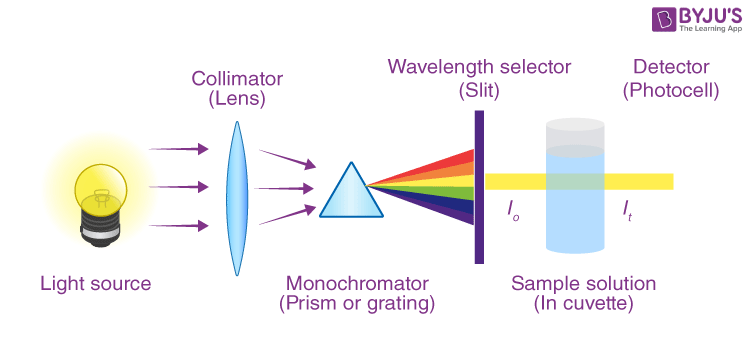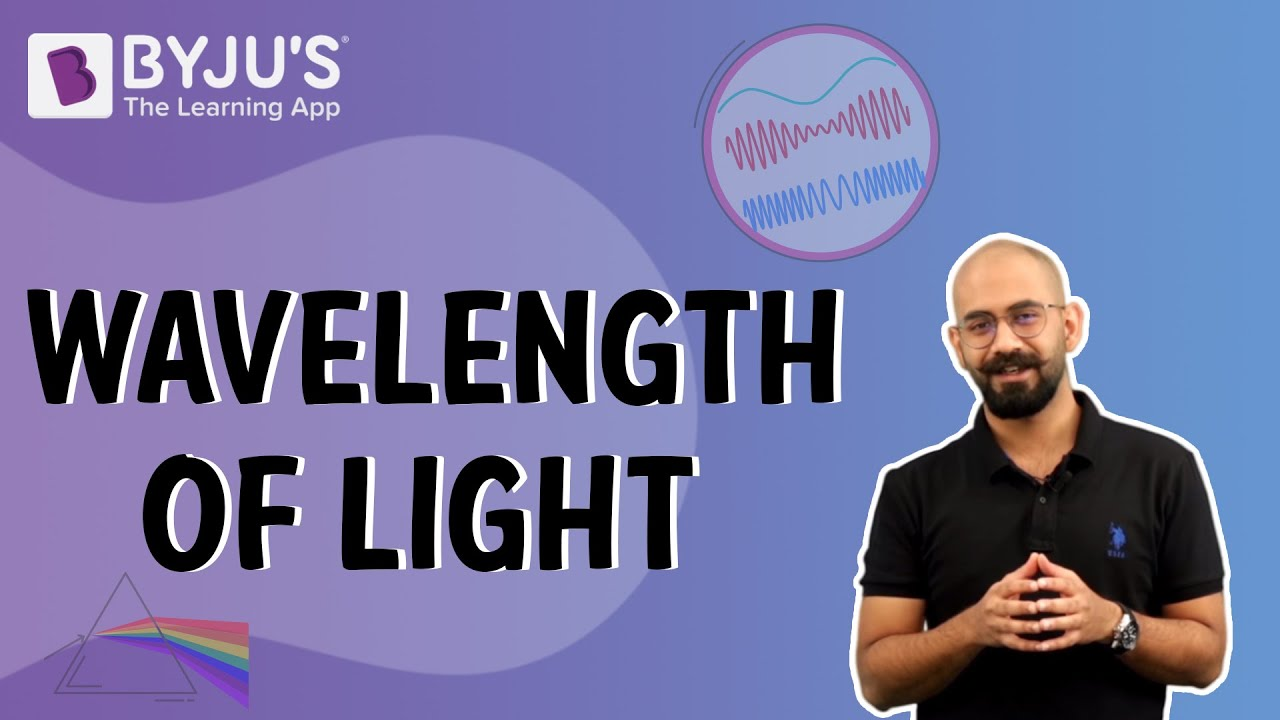# Optical Density

Optical density is the process of transmission of light or other electromagnetic radiation by matter. The process of emission and absorption depends on the wavelength of the radiations, which includes the interaction between fundamental particles like electrons, atoms, ions, etc.

When a beam of light interacts with absorbing atoms, absorption takes place. It depends on the sample’s thickness and the concentration of the absorbing atoms.

## Optical Density Definition

It is often said to be identical to the absorbance. It is a logarithmic ratio of the falling radiation to the transmitted radiation through a material.

For a  given wavelength, the expression of optical element transmittance is expressed as:

Log10 (1/T)

Where T is transmittance.

### Few things to note:

• The higher the optical density, the lower the transmittance.
• The ten times of this density is equal to transmission loss expressed in decibels. For example, the density of 0.3 corresponds to a transmission loss of 3dB.

## Optical Density Measurement

The measurement is done at maxima of the absorbance spectra as there is the least chance of absorbance with the change in the wavelength. The measurement is a common method to quantify various important parameters like concentration of cell, production of biomass, and much more.

$$\begin{array}{l}\large Absorbance\; A = -log \left ( \frac{I}{I_{0}} \right )\end{array}$$

where,

I is the intensity of light that passes through the sample

I0 is the initial light intensity.## Optical Density of Spectrophotometer

The formula for spectrophotometer is:

$$\begin{array}{l}\large OD = \frac{A}{L}\end{array}$$

Where,

L = the thickness of the sample.

## Watch the video and understand the relationship between frequency and wavelength.Stay tuned with BYJU’S to learn more about electromagnets, the atom, the wavelength of light, and many more.

Test your Knowledge on Optical Density